×

Search anything:

# Technical Introduction to Quantum Computing

#### Software EngineeringFREE BOOK -> Problems for the day before your Coding Interview (on Amazon)

The popular feline Schrödinger's cat which is in the state of being alive and dead at simultaneously in the box involves the paradoxical world of quantum in it. We all can agree that whenever quantum gets associated there are unexpected and magical things that tend to take place. The new era sees the revolution in the world of computing with incorporation of the quantum phenomena.

## Quantum in Computing?

The mixing of a complex spectacles like quantum with something as necessary as coding and computers has opened a new sphere full of possibilities and opportunities. Quantum Computing is the trending technologies which is in spite being under development has shown tremendous potential.

It shows promise on fields like medicine, artificial intelligence, machine learning, algorithm solving and much more. It's a miracle in building.

## Structure of a Quantum Computer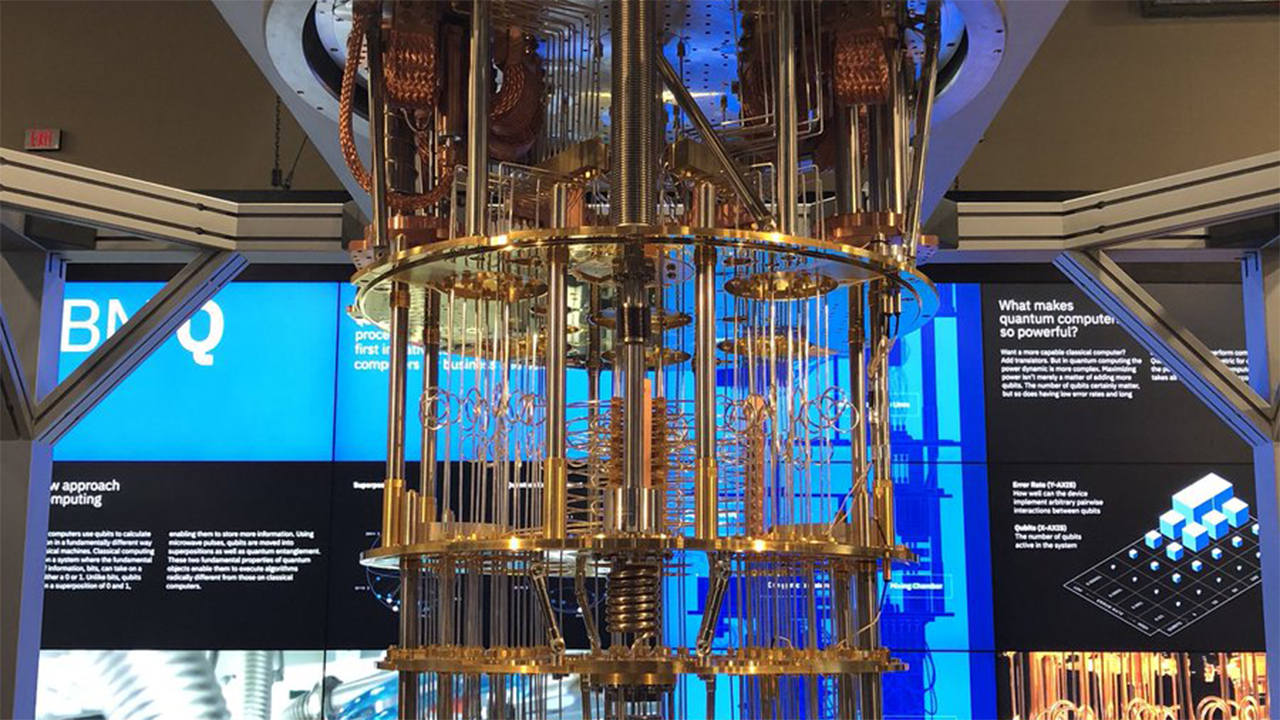This is what a quantum computer looks. It is a 50-qubit chip computer built by IBM. Something out of the ordinary.

The classical and quantum have several similarities and differences.

### The Quantum Transistor

The classical computer uses transistors to have a high (1) or low (0) voltages and store information, the quantum computer uses quantum mechanics to achieve something similar.

The quantum field effect transistor (QFET) is a MOSFET which uses quantum phenomenon of propagating waveform through a barrier. By doing so the the speed of transistor increases 10x and power consumption decreases 10x.
These MOSFETs are also made of dielectric materials.

### Quantum Gates

Just like classical gates make up the digital circuits, quantum gates are the building blocks of quantum computer. Quantum logic gates have the ability to perform all the logical operation performed by classical logic gates and are also reversible in nature.

They are represented by unitary matrices.These are two orthogonal matrices.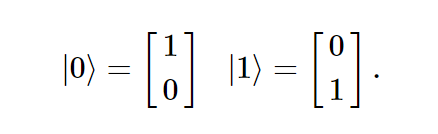These are the quantum logical gates. Combination of these gates makes circuit composition.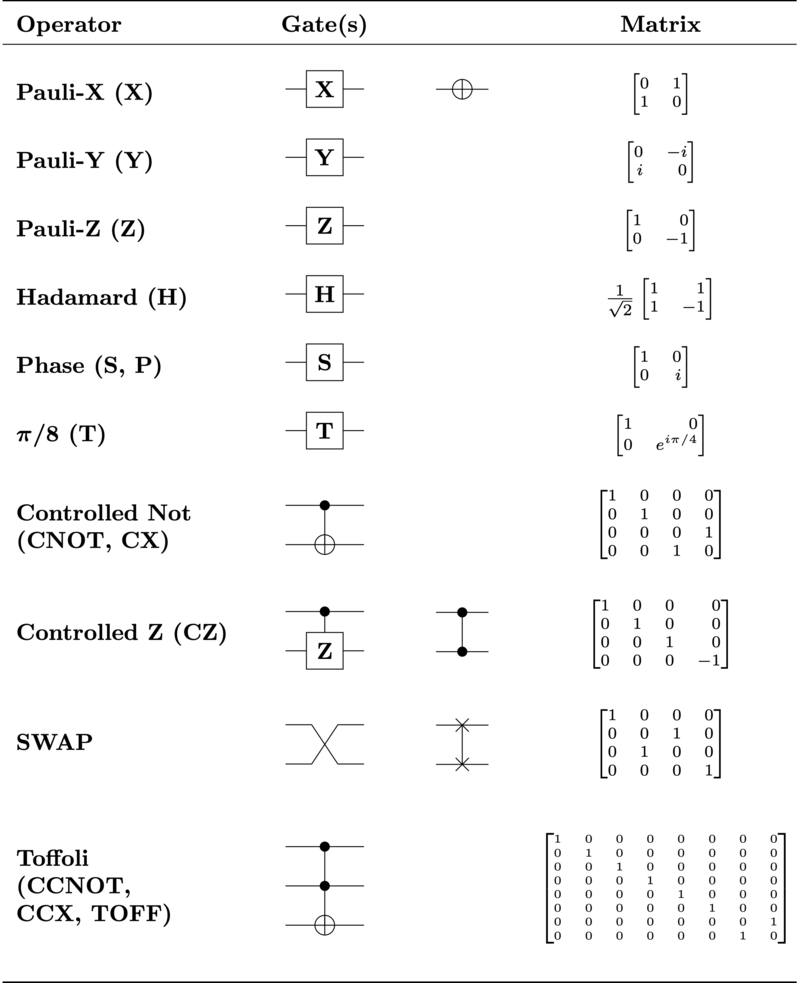Some of the important quantum gates:

It acts on a single qubit by creating a superposition. Several quantum gates make use of Hadamard transform through the gate. It is used to produce 0 or 1 by applying equal probability.

• Toffoli (CCNOT) gate
Also known as Deutsch Gate is a 3-bit gate used in classical computer, it is also used in quantum computation. It is a controlled gate.

## The Quantum Phenomena ft. Superposition and Entanglement:

The quantum phenomena used in computing includes the concept of superposition and entanglement.

Quantum superposition refers to the existence of several discrete states at the same time. Such remarkable characteristic allows flexibility and qualities which makes quantum computing so interesting.
Example of quantum superposition: In quantum state; electron has spin up and spin down simultaneously.

### The Qubit State:

The Qubit or the quantum bit is the unit of quantum information used in quantum computing.∣0⟩ and ∣1⟩ are the two level states of a single qubit. Due to the involvement of superposition the qubit can be in either of the state or both.
The qubit is much more advanced than its classical counterpart bit as it is able to store much more information.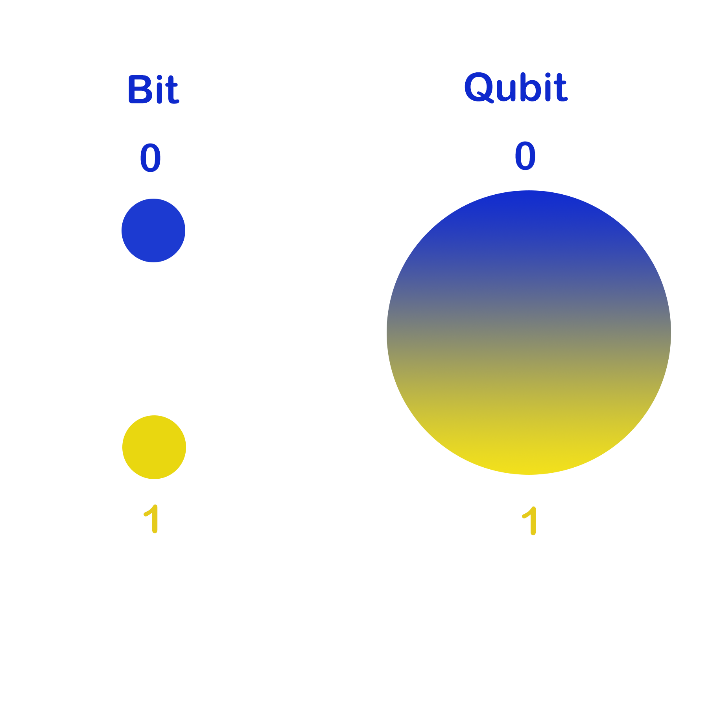Quantum Entanglement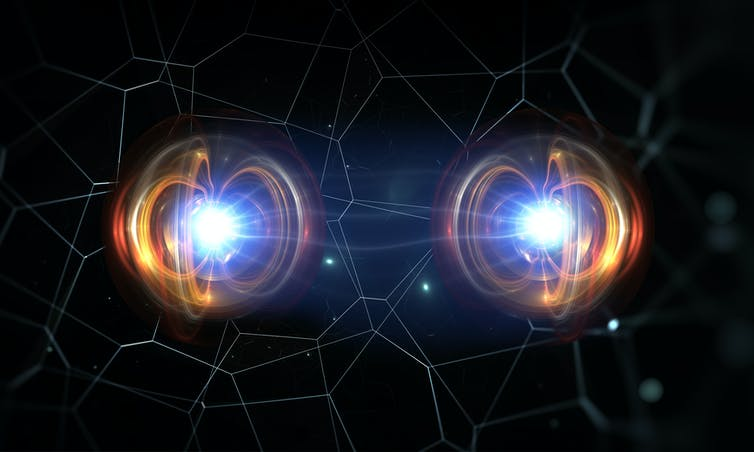The quantum state in which all the particles of a group are entangled and interact such that each particle of the group cannot be described independently of other particles is the phenomenon of quantum entanglement. It should be noted this is irrespective of the distance between the two particles.
In Albert Einstein's own words entanglement can be called “spooky action at a distance.”
Example of quantum Entanglement: When the total spin of the system is zero and the spin of one of the particles is up then it can be assured that the other particle's spin is down. This complementary relationship seems quite obvious however in quantum mechanics such a connection arises the probability distribution.
Entanglement of qubits provides quantum computer the upper hand than the classical computer.

## Algorithms and Quantum

Just like in classical computers an algorithm is group of sequential steps to solve a problem quantum algorithm refers to the set of steps which are used by quantum computers to solve a problem.
It should be noted that quantum computers can solve all the algorithms which can be solved by classical computers.

• Shor's Algorithm:
This quantum algorithm find's its use in the process of integer factorization. Integer factorization is the process of dividing a given integer into smaller integers. Shor's Algorithm which is proposed by Peter Shor is amongst the best known algorithm in the field factoring. The algorithm runs drastically faster than the classical algorithm for factoring.

Shor's algorithm achieves factorization with polynomial complexity unlike classical algorithm which requires superpolynomial complexity.
A peek into Shor's!
The catch about Shor's is that it uses order finding problem than the factorization.

Division Use
Classical Part: Reduction to order finding problem
Quantum Part: Efficient use of Quantum fourier transform
Application of quantum algorithm for order-finding

Here is the quantum subroutine of Shor's: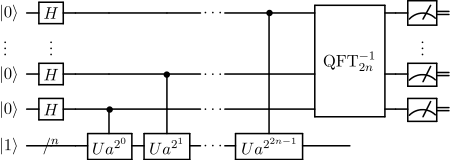The Period Function used in Shor here a, N are positive integers with no common factors and a < N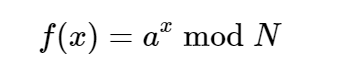A unitary operation takes place which finds out the eigen values. These eigen values are calculated using phase estimation algorithm.

For phase estimation a quantum gate is build using an arrangement of reversible gates like Toffoli Gates.

Here is how the circut, state of eigen values, Q-sphere look like in IBM Circuit Composer where a=7 and N=15.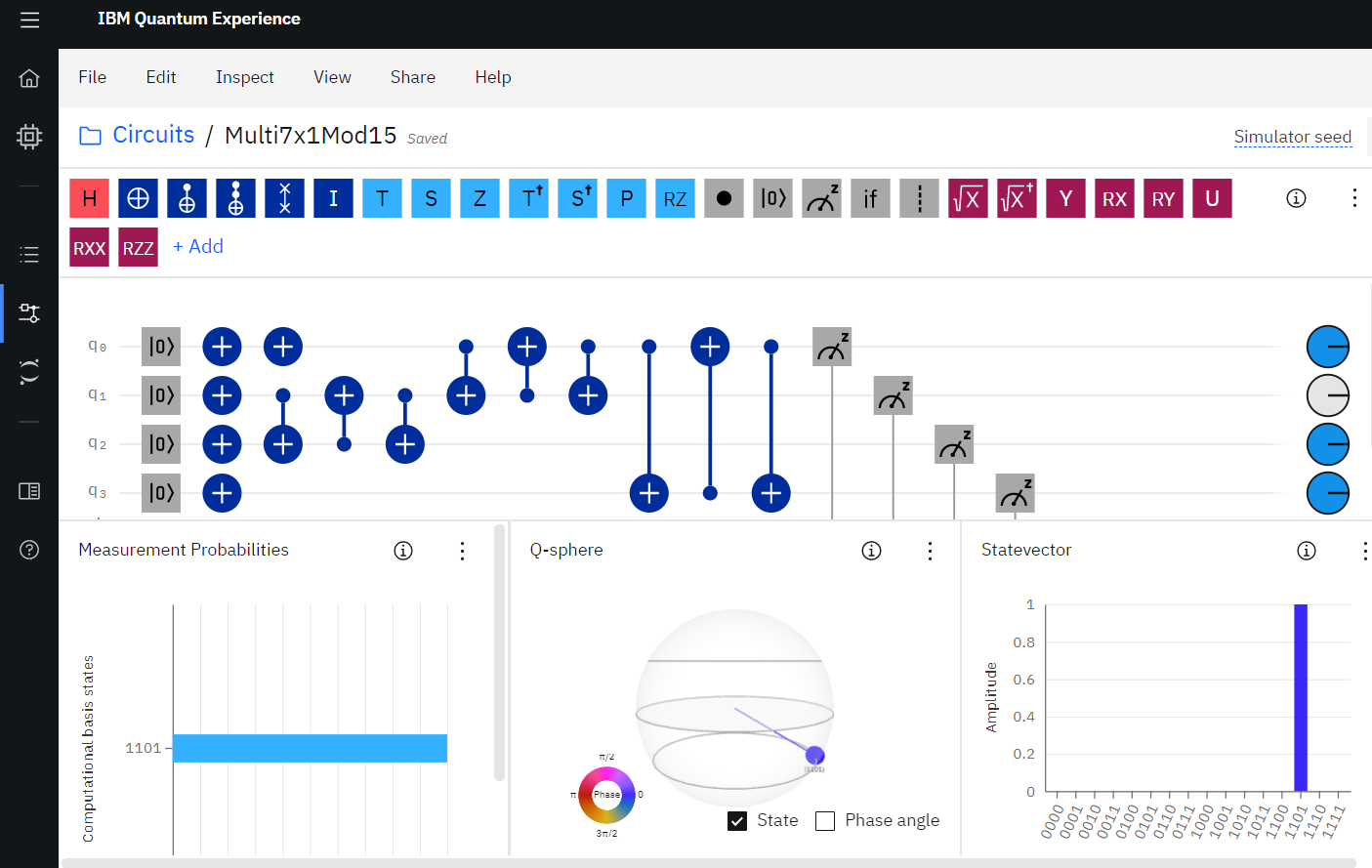https://quantum-computing.ibm.com/
IBM Quantum Experience provides an excellent platform to learn, code and understand the quantum world. Clink on the link above to know more.

• Grover's Algorithm:
This quantum algorithm is used when dealing with probability. The algorithm devised by Lev Grover finds its application with unstructured database. In the statistical realm the Grover's algorithm is used to find the mean and medium of given numbers.
An unsorted database requires O(N) time i.e. linear time for search however Grover takes O(√N) which is faster than its classical counterpart.

How Grover works?
The working of the algorithm uses qubit's superposition using quantum gates. Implementation of oracle

Process Working
Qubit's Superposition This happens using Quantum Gates
Oracle Implementation by phase flip
Measurement Variation in Quantum Circuits and measuring the qubits

Here is the circuit representation: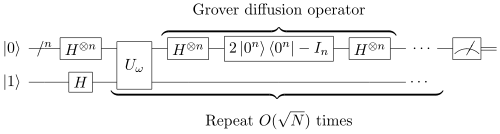Quantum World and it's Possibilities:
There is a promising future that quantum computing shows and which might change the way we live. Advancements in technology are absolute and this is what makes science and technology beautiful.

It would not be surprising to see quantum computing effecting sectors of finance, defence, cyber security and what not.

When something as powerful as quantum is worked upon it one can expect wonders.

“A classical computation is like a solo voice—one line of pure tones succeeding each other. A quantum computation is like a symphony—many lines of tones interfering with one another.”
― Seth Lloyd#### Anjali Chaturvedi

Student IIIT-Allahabad. Trying to figure out the monster group, monstrous moonshine, and quarks.

Technical Introduction to Quantum Computing